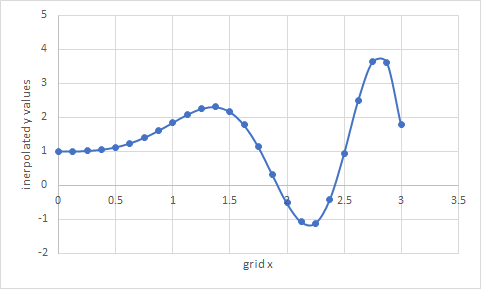# =INTERPXY(x, y, q, [options])

INTERPXY is a versatile 2D interpolation function based on splines.

Use INTERPXY to interpolate from a set of (x,y) data points at an arbitrary point.
Use INTERPXY to map a scattered (x,y) data points onto a uniform grid for easy plotting in Excel.

With optional arguments, you can control the interpolating spline properties.

INTERPXY automatically sorts your data points and averages the y values if your data set contains duplicate x points.

### Required Inputs

x x-coordinates of the scattered (x,y) points.

y corresponding y values.

q query point to interpolate at.

If q is a vector of points, run INTERPXY as an array formula.

### Optional Inputs

ctrl a set of key/value pairs for algorithmic control as detailed below.

Description of key/value pairs for algorithmic control

 Key ORDER Admissible Values (Integer) 1 or 3 (linear or cubic) Default Value 3
 Keys ISLOPE, ESLOPE Admissible Values real number Default Value Unconstrained Remarks If defined, the spline curve first derivative will match the supplied value.Cannot be imposed with a linear spline or with PERIOIDC option enabled.
 Key PERIODIC Admissible Values (Boolean) True or False Default Value False Remarks The y-values at the end points must be equal if PERIOIDC is set to True.ISLOPE, ESLOPE constraints are ignored
 Key SFACTOR Admissible Values (real) ≥ 0 Default Value 0 Remarks A zero value will compute an exact interpolating spline passing through the data points. A large value will compute a least-squares smooth fitting curve.The smoothing factor has a significant effect on the computed integral value.

w strictly-positive corresponding set of weights for the (x,y) data points. Default value is unity.

In this example, we sample the function $f\left(x\right)=xsin\left({x}^{2}\right)+1$ at random points, and then reconstruct the function at a uniform grid for easy plotting in Excel.

Using Excel's RAND() function we generate a vector of random numbers in the range [0 to 3]. To preserve the generated random numbers from continuously changing, we copy them into range A4:A28 as numbers only. Using AutoFill, we generate corresponding y values in range B4:B28 using the formula =A4*SIN(A4^2)+1. Next we generate a uniform grid for x values in range D4:D28 from 0 to 3 in increment of 0.125.
Finally, we evaluate =INTERPXY(A4:A28, B4:B28, D4:D28) as array formula in range E4:E28 to interpolate the scattered data onto the uniform grid. The numerical values are shown below.

 A B D E 3 scattered x y values unifrom x interpolated y 4 1.753029064 1.119956695 0 1.001328093 5 0.568116228 1.180195958 0.125 1.002172559 6 1.440216258 2.26153531 0.25 1.01561687 7 2.28511224 -0.995044984 0.375 1.052557644 8 1.015003489 1.870282715 0.5 1.123729162 9 1.217131211 2.212271884 0.625 1.238002939 10 2.429384636 0.096014392 0.75 1.400044332 11 1.061113672 1.95784828 0.875 1.606510771 12 2.828238912 3.79857802 1 1.84148167 13 1.590985661 1.911889769 1.125 2.073236147 14 1.454578337 2.243848266 1.25 2.249813639 15 1.639359184 1.719102308 1.375 2.305014902 16 2.385193008 -0.335023914 1.5 2.166955797 17 0.955015097 1.755190226 1.625 1.78044105 18 0.32744209 1.035040565 1.75 1.138439619 19 1.579686587 1.951197701 1.875 0.303724928 20 0.414115731 1.070669882 2 -0.514903922 21 0.913884208 1.677572239 2.125 -1.068933993 22 0.265305572 1.01865866 2.25 -1.109851826 23 1.619483831 1.803093365 2.375 -0.422949211 24 1.610414434 1.839212722 2.5 0.926799955 25 2.658802449 2.881273233 2.625 2.50361038 26 2.310702177 -0.871246759 2.75 3.629673183 27 2.79968645 3.799339763 2.875 3.616506327 28 0.729757139 1.370518104 3 1.774219949

We can also generate column E using AutoFill instead of evaluating INTERPXY as array formula. However, if we use the AutoFill feature, we must lock arguments 1 and 2 so Excel does not increment them during the AutoFill by using the formula =INTERPXY(A$4:A$28, B$4:B$28, D4). The array formula option is computationally more efficient.

The Figure below shows the interpolated y values plotted against the generated uniform x values. This plot is easily generated in Excel by highlighting columns D and E and inserting a scatter plot.INTERPXY computes the interpolation by fitting a spline model to the data.

Question or Comment? Email us:
support @ excel-works.comExceLab: Transforming Excel into a Calculus Power House

ExceLab functions and methods are protected by USA Patents 10628634, 10114812, 9892108 and 9286286.